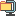검색어 입력폼

# DSP 디지털 신호처리 FFT

저작시기 2009.06 |등록일 2009.06.21압축파일 (zip) | 4페이지 | 가격 2,500원

## 소개글

Homework (Matlab#3)

## 목차

Homework (Matlab#3)

## 본문내용

Homework (Matlab#3)
Due day : Dec. 10

In Matlab command, load the data file KTXSignal`. Check the data variable. The sampling rate of the signal is 100 samples/sec.

1. Perform DFT for the KTXSignal using `FFT`. Plot the spectrum magnitude for KTXSignal. Since the DFT is discrete, you should use the plot function stem.
>> stem(abs(FFT(KTXSignal)))

2. Plot the spectrum magnitude along the normalized frequency .
>> stem(, abs(FFT(KTXSignal)))

3. Plot the spectrum magnitude along the normalized actual frequency `f`.
>> stem(f, abs(FFT(KTXSignal)))

4. Determine the signal power at the frequency range . (* The equation for the signal power is .*)

없음

KTXSignal.mat
Matlab(HW3).hwp
Untitled1.m
1.jpg
DSP matlab#3.hwp